﻿ Remap Intensity | Sample Dialogs | Imaging Sample Common Dialogs Help
In This Topic ▼

Remap Intensity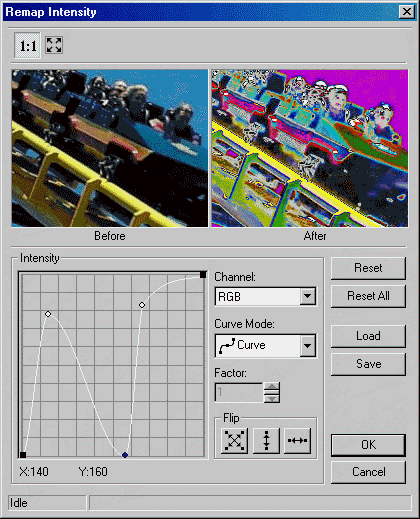This dialog has the following fields:

Channel Drop-down list box:

Use this to select the channel to remap. There are four choices:

• RGB: remap all channels.
• Red: remap the red channel.
• Green: remap the green channel.
• Blue: remap the blue channel.

Curve Mode Drop-down list box:

Use this to select the curve mode to use when remapping. There are four choices:

•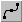Curve: remaps the selected channel using curve data.

•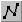Linear: remaps the selected channel using linear data.

•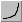Exponential: remaps the selected channel using exponential data, which is affected by the Factor value.

•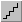Logarithm: remaps the selected channel using logarithmic data, which is affected by the Factor value.

Factor Edit box:

Use this to set the factor of the Exponential/Logarithm curve. Enter the value directly in the edit box or use the arrows to set the desired value.

Flip Buttons:

Use these buttons to flip the curve if the Curve Mode is either Curve or Linear. The following options are available:

•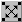Both. Flip the curve vertically and horizontally.

•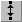Vertically. Flip the curve vertically.

•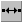Horizontally. Flip the curve horizontally.

Reset Button:

Use the Reset button to set the curve of the current channel to default values.

Reset All Button:

Use the Reset All button to set the curve for all the channels to default values.

Use the Load button to load an "*.lri" file that contains Remap Intensity curve settings, using the Load dialog. The loaded file will set a new curve for each channel.

✎ NOTE